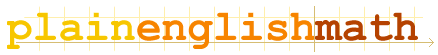You are here: Mathematics → Glossary

# The Plain English Math Glossary

symbols | A | B | C | D | E | F | G | H | I | J | K | L | M | N | O | P | Q | R | S | T | U | V | W | X | Y | Z

## symbols

α
The lowercase Greek letter alpha. (Uppercase α is written as A)
β
The lowercase Greek letter beta.
δ
The lowercase Greek letter delta. In calculus, this represents an infinitesimally small change in a variable, for example δx
The symbol for integration in calculus.
||
Shorthand for "parallel"
π
The lowercase Greek letter pi. The constant π is equal to approximately 3.14, which is the angle 180° in radians.

## C

Circumference
The distance around the perimeter of a circle - the distance you'd measure if you could straighten out the edge of a circle and hold it up against a ruler.

## D

Derivative
A function that represents the rate of change of another function – how much a function's value changes as its parameters change.
Differentiation
The process of finding the derivative of a function.

## P

Parallel
Describes a line, vector, etc. pointing in exactly the same direction as another line, vector, etc. (Shorthand: || )
Perpendicular
Describes a line, vector, etc. pointing exactly 90° to another, such that the two lines would form a cross at right angles if drawn on top of each other.

## R

The angle you'd cover by moving around the edge of any circle a distance equal to its radius.
The distance from the center of a circle to its edge.

## U

Unity
A word meaning "equal to 1," or simply the number 1. ("Unity" = 1 unit)

## W

Wavelength
The length of a single cycle of a wave, such as a sine wave. This is the distance afterwhich a wave returns to its starting point and repeats itself.
Wavenumber
In the case of a sine wave; the number of wave cycles that fit into a period of 2π. For example, a wave with a wavelength of π has a wavenumber of 2, because precisely 2 wave cycles (of length π) are needed to span a distance of 2π. Wavenumber is equal to 2π/λ, where λ is the wavelength.

Fundamentals

Vectors

Matrices

Circles and Triangles

Complex Numbers

Sequences and Series

Calculus

Appendices

Glossary